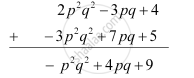Share

# Add the Following 2psquare2qsquare2 − 3pq + 4, 5 + 7pq − 3squarep2qsquare2 - CBSE Class 8 - Mathematics

ConceptAddition and Subtraction of Algebraic Expressions

#### Question

Add the following 2p2q2 − 3pq + 4, 5 + 7pq − 3p2q2

#### Solution

The given expressions written in separate rows, with like terms one below the other and then the addition of these expressions are as follows.Thus, the sum of the given expressions is −p2q2 + 4pq + 9.

Is there an error in this question or solution?

#### APPEARS IN

NCERT Solution for Mathematics Textbook for Class 8 (2018 to Current)
Chapter 9: Algebraic Expressions and Identities
Ex. 9.10 | Q: 3.3 | Page no. 140
Solution Add the Following 2psquare2qsquare2 − 3pq + 4, 5 + 7pq − 3squarep2qsquare2 Concept: Addition and Subtraction of Algebraic Expressions.
S